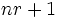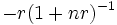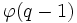# Central automorphism group of general linear group

## Definition

### General definition

Let$k$ be a field and$n$ be a natural number. The central automorphism group of general linear group, or inner-centralizing automorphism group of general linear group, is defined as the group of automorphisms of the general linear group$GL(n,k)$ the form:$A \mapsto A\varphi(\det A)$

where$\varphi$ is a homomorphism from the multiplicative group of$k$ to itself, such that the map$x \mapsto x\varphi(x^n)$ is an automorphism from the multiplicative group of$k$ to itself.

We denote this group by$\operatorname{CAut}(GL(n,k))$.

Note that this is precisely the group of inner-centralizing automorphisms of the general linear group, i.e., the automorphisms that commute with all the inner automorphisms, or equivalently, that induce the identity map on the projective general linear group. It is thus the kernel of the homomorphism$\operatorname{Aut}(GL(n,k)) \to \operatorname{Aut}(PGL(n,k))$. This group also turns out to be the center of the automorphism group of$GL(n,k)$.

## The case of a finite field

For a finite field with$q$ elements, all such automorphisms are of the form:$A \mapsto A (\det A)^r$.

where$r$ is some positive integer with the property that$nr + 1$ is relatively prime to$q - 1$.$r$ is viewed modulo$q - 1$: two values of$r$ yield the same automorphism iff they are congruent modulo$q - 1$.

The composite of the automorphism corresponding to$r$ and the automorphism corresponding to$s$ is the automorphism corresponding to$r + s + nrs$. The inverse of the automorphism correspond to$r$ is the automorphism corresponding to$-r(1 + nr)^{-1}$, where the inverse is taken modulo$q - 1$.

### The nature and size of this group

When$n$ is relatively prime to$q$, we can identify this with the automorphism group of the multiplicative group of$k$, because the permissible$r$s correspond uniquely to the values$nr + 1$ that are relatively prime to$q - 1$, and the composition of these works like multiplying the corresponding$nr + 1$. In particular, this is an abelian group of order$\varphi(q - 1)$, where$\varphi$ is the Euler phi-function. In the case that there is a gcd$d$, the abelian group has size$(d'/d) \varphi((q - 1)/d')$ where$d'$ is the largest divisor of$q - 1$ that contains only those prime divisors of$q - 1$ that are already divisors of$d$.